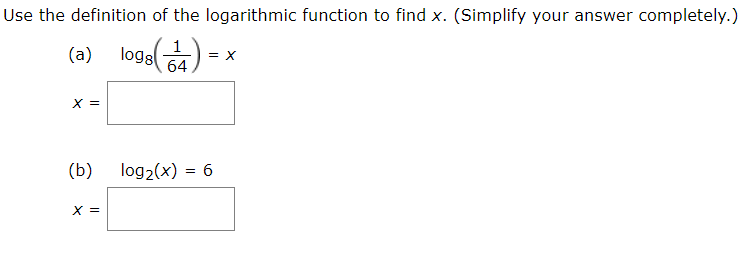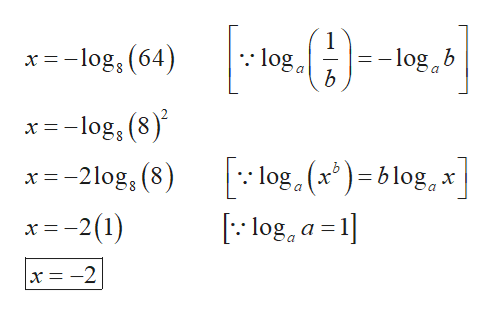# Use the definition of the logarithmic function to find x. (Simplify your answer completely.)oge(7)logs(a)o0)х 3(b)log2(x) = 6

Question
1 viewshelp_outlineImage TranscriptioncloseUse the definition of the logarithmic function to find x. (Simplify your answer completely.) oge(7) logs (a) o0) х 3 (b) log2(x) = 6 fullscreen
check_circle

Step 1

(a) The logarithmic equation is,

Step 2

Apply logarithm...help_outlineImage Transcriptionclose:: loga x =-log, (64) - log b x = -log, (8) log. (x*) =b log, x [:: log, a =1] x =-2log, (8) x=-2(1) x= -2 fullscreen

### Want to see the full answer?

See Solution

#### Want to see this answer and more?

Solutions are written by subject experts who are available 24/7. Questions are typically answered within 1 hour.*

See Solution
*Response times may vary by subject and question.
Tagged in
MathCalculus

### Other